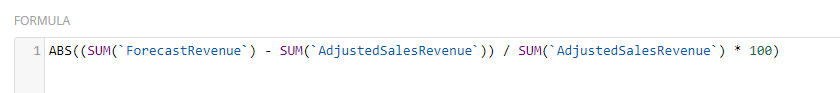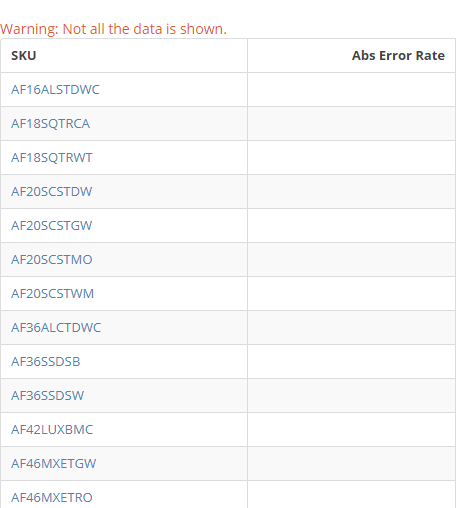Replace Undefined/Blank with a value in Beast Mode

Hi,

I am calculating accuracy with a formula inside Beast Mode:

(A - F) / A * 100.There are few instances where the denominator is 0.

For those instances, I am getting blank values as shown below:I want to replace the blank/undefined with a different value

I tried to use the CASE and IFNULL BeastMode functions but that did not work.

Can someone help me with this?

Thanks,

Prajwal

• Here's what I've done in the past that has worked.  Call out the denominator as zero calulcation first, then go on to the calculation if it's fine.

CASE

WHEN IFNULL(SUM(`AdjustedSalesRevenue),0) = 0 THEN 0

ELSE

[calculation]

END

Does something like that work for you?